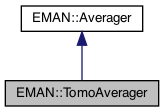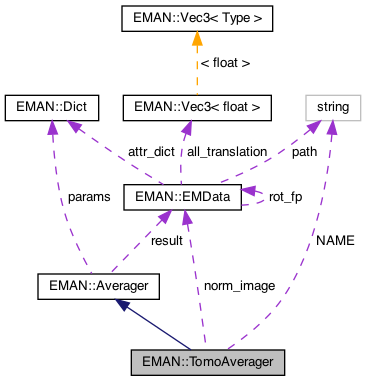EMAN2
EMAN::TomoAverager Class Reference

TomoAverager averages a list of volumes in Fourier space. More...

`#include <averager.h>`

Inheritance diagram for EMAN::TomoAverager:[legend]
Collaboration diagram for EMAN::TomoAverager:[legend]

## Public Member Functions

TomoAverager ()

virtual ~TomoAverager ()

To add an image to the Averager. More...

EMDatafinish ()
Finish up the averaging and return the result. More...

string get_name () const
Get the Averager's name. More...

string get_desc () const

TypeDict get_param_types () const
Get Averager parameter information in a dictionary. More...

virtual void mult (const float &)
Multiply the result image by some floating point constant This is useful when weighting the input images prior to calling add_image - a situation where it is likely you want to divide by the sum of the weights. More...Public Member Functions inherited from EMAN::Averager
Averager ()

virtual ~Averager ()

virtual void add_image_list (const vector< EMData * > &images)
To add multiple images to the Averager. More...

virtual void set_params (const Dict &new_params)
Set the Averager parameters using a key/value dictionary. More...

## Static Public Member Functions

static AveragerNEW ()

## Static Public Attributes

static const string NAME = "mean.tomo"

## Private Attributes

EMDatanorm_image

float thresh_sigma

float overlap

int nimgProtected Attributes inherited from EMAN::Averager
Dict params

EMDataresult

## Detailed Description

TomoAverager averages a list of volumes in Fourier space.

It excludes values near zero from the average, assuming they are part of the missing-cone/wedge. A threshold

Parameters
 thresh_sigma a number, multiplied by the standard deviation of the image, below-which values are considered zero

Definition at line 345 of file averager.h.

## ◆ TomoAverager()

 TomoAverager::TomoAverager ( )

Definition at line 93 of file averager.cpp.

94 : norm_image(0),nimg(0),overlap(0)
95{
96
97}
EMData * norm_image
Definition: averager.h:389

Referenced by NEW().

## ◆ ~TomoAverager()

 virtual EMAN::TomoAverager::~TomoAverager ( )
inlinevirtual

Definition at line 349 of file averager.h.

350 {
351 if (norm_image!=0) delete norm_image;
352 if (result!=0) delete result;
353 }
EMData * result
Definition: averager.h:158

References norm_image, and EMAN::Averager::result.

## Member Function Documentation

 void TomoAverager::add_image ( EMData * image )
virtual

To add an image to the Averager.

This image will be averaged in this function.

Parameters
 image The image to be averaged.

Reimplemented from EMAN::Averager.

Definition at line 99 of file averager.cpp.

100{
101 if (!image) {
102 return;
103 }
104
105 if (!image->is_complex()) {
106 image=image->do_fft();
107 image->set_attr("free_me",(int)1);
108 }
109
110 if (result!=0 && !EMUtil::is_same_size(image, result)) {
111 LOGERR("%s Averager can only process same-size Images",
112 get_name().c_str());
113 return;
114 }
115
116 int nx = image->get_xsize();
117 int ny = image->get_ysize();
118 int nz = image->get_zsize();
119// size_t image_size = (size_t)nx * ny * nz;
120
121 if (norm_image == 0) {
122// printf("init average %d %d %d",nx,ny,nz);
124 result->to_zero();
125
127 norm_image->to_zero();
128
129 thresh_sigma = (float)params.set_default("thresh_sigma", 0.5);
130 overlap=0.0f;
131 nimg=0;
132 }
133
134 float *result_data = result->get_data();
135 float *norm_data = norm_image->get_data();
136 float *data = image->get_data();
137
138 vector<float> threshv;
140 for (int i=0; i<nx/2; i++) threshv[i]*=threshv[i]*thresh_sigma; // The value here is amplitude, we square to make comparison less expensive
141
142 size_t j=0;
143 // Add any values above threshold to the result image, and add 1 to the corresponding pixels in the norm image
144 int k=0;
145 for (int z=0; z<nz; z++) {
146 for (int y=0; y<ny; y++) {
147 for (int x=0; x<nx; x+=2, j+=2) {
148 float rf=Util::hypot3(x/2,y<ny/2?y:ny-y,z<nz/2?z:nz-z); // origin at 0,0; periodic
149 int r=int(rf);
150 if (r>ny/2) continue;
151 float f=data[j]; // real
152 float g=data[j+1]; // imag
153 float inten=f*f+g*g;
154
155 if (inten<threshv[r]) continue;
156
157 k+=1;
158 result_data[j] +=f;
159 result_data[j+1]+=g;
160
161 norm_data[j] +=1.0;
162 norm_data[j+1]+=1.0;
163 }
164 }
165 }
166// printf("%d %d\n",k,nx*ny*nz);
167 overlap+=(float)k/(nx*ny*nz);
168 nimg++;
169
170 if (image->has_attr("free_me")) delete image;
171}
type set_default(const string &key, type val)
Default setting behavior This can be achieved using a template - d.woolford Jan 2008 (before there wa...
Definition: emobject.h:569
vector< float > calc_radial_dist(int n, float x0, float dx, int inten)
Definition: emdata.cpp:2781
static bool is_same_size(const EMData *image1, const EMData *image2)
Check whether two EMData images are of the same size.
Definition: emutil.cpp:1224
string get_name() const
Get the Averager's name.
Definition: averager.h:358
static float hypot3(int x, int y, int z)
Euclidean distance function in 3D: f(x,y,z) = sqrt(x*x + y*y + z*z);.
Definition: util.h:827
#define LOGERR
Definition: log.h:51
#define y(i, j)
Definition: projector.cpp:1516
#define x(i)
Definition: projector.cpp:1517

## ◆ finish()

 EMData * TomoAverager::finish ( )
virtual

Finish up the averaging and return the result.

Returns
The averaged image.

Implements EMAN::Averager.

Definition at line 173 of file averager.cpp.

174{
175 if (norm_image==0 || result==0 || nimg==0) return NULL;
176
177 int nx = result->get_xsize();
178 int ny = result->get_ysize();
179 int nz = result->get_zsize();
180 size_t image_size = (size_t)nx * ny * nz;
181
182 float *result_data = result->get_data();
183 float *norm_data = norm_image->get_data();
184
185// printf("finish average %d %d %d",nx,ny,nz);
186 // normalize the average
187 for (size_t j = 0; j < image_size; j++) {
188 if (norm_data[j]==0.0) result_data[j]=0.0;
189 else result_data[j]/=norm_data[j];
190 }
191
192 norm_image->update();
193 result->update();
194
195 EMData *ret;
196 if ((int)params.set_default("doift", 1))
197 ret = result->do_ift();
198 else
199 ret = result->copy();
200
201 ret->set_attr("ptcl_repr",norm_image->get_attr("maximum"));
202 ret->set_attr("mean_coverage",(float)(overlap/nimg));
203 if ((int)params.set_default("save_norm", 0))
204 norm_image->write_image("norm.hdf");
205
206 if (params.has_key("normout")) {
207 EMData *normout=(EMData*) params["normout"];
208 normout->set_data(norm_image->copy()->get_data());
209 normout->update();
210 }
211
212 delete result;
213 delete norm_image;
214 result=0;
215 norm_image=0;
216
217 return ret;
218}
bool has_key(const string &key) const
Ask the Dictionary if it as a particular key.
Definition: emobject.h:511
EMData stores an image's data and defines core image processing routines.
Definition: emdata.h:82

## ◆ get_desc()

 string EMAN::TomoAverager::get_desc ( ) const
inlinevirtual

Implements EMAN::Averager.

Definition at line 363 of file averager.h.

364 {
365 return "Average of volumes in Fourier space, excluding any pixels with near 0 intensity.";
366 }

## ◆ get_name()

 string EMAN::TomoAverager::get_name ( ) const
inlinevirtual

Get the Averager's name.

Each Averager is identified by a unique name.

Returns
The Averager's name.

Implements EMAN::Averager.

Definition at line 358 of file averager.h.

359 {
360 return NAME;
361 }
static const string NAME
Definition: averager.h:386

References NAME.

## ◆ get_param_types()

 TypeDict EMAN::TomoAverager::get_param_types ( ) const
inlinevirtual

Get Averager parameter information in a dictionary.

Each parameter has one record in the dictionary. Each record contains its name, data-type, and description.

Returns
A dictionary containing the parameter info.

Reimplemented from EMAN::Averager.

Definition at line 373 of file averager.h.

374 {
375 TypeDict d;
376 d.put("thresh_sigma", EMObject::FLOAT, "multiplied by the standard deviation of the image, below-which values are considered zero. Default = .01");
377 d.put("save_norm", EMObject::INT, "If set, will save the normalization volume as norm.hdf. Mainly for debugging purposes.");
378 d.put("normout", EMObject::EMDATA, "If set, will save the normalization volume in the given EMData object.");
379 d.put("doift", EMObject::INT, "IFT the resulting volume. Default is 1.");
380
381 return d;
382 }

## ◆ mult()

 virtual void EMAN::TomoAverager::mult ( const float & s )
inlinevirtual

Multiply the result image by some floating point constant This is useful when weighting the input images prior to calling add_image - a situation where it is likely you want to divide by the sum of the weights.

Hence call mult after all of the weighted images have been added.

Parameters
 s the scaling factor.
Exceptions
 NullPointerException if the EMData pointer (result) is NULL

Reimplemented from EMAN::Averager.

Definition at line 384 of file averager.h.

384{ }

## ◆ NEW()

 static Averager * EMAN::TomoAverager::NEW ( )
inlinestatic

Definition at line 368 of file averager.h.

369 {
370 return new TomoAverager();
371 }

References TomoAverager().

## ◆ NAME

 const string TomoAverager::NAME = "mean.tomo"
static

Definition at line 386 of file averager.h.

Referenced by get_name().

## ◆ nimg

 int EMAN::TomoAverager::nimg
private

Definition at line 392 of file averager.h.

## ◆ norm_image

 EMData* EMAN::TomoAverager::norm_image
private

Definition at line 389 of file averager.h.

Referenced by add_image(), finish(), and ~TomoAverager().

## ◆ overlap

 float EMAN::TomoAverager::overlap
private

Definition at line 391 of file averager.h.